# Smirnov class

(diff) ← Older revision | Latest revision (diff) | Newer revision → (diff)

The set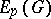of all functionsholomorphic in a simply-connected domain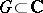with rectifiable Jordan boundary, such that for every function in it there is a sequence of closed rectifiable Jordan curves,with the following properties:

1)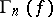tends toasin the sense that if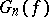is the bounded domain with boundary, then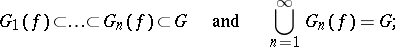2)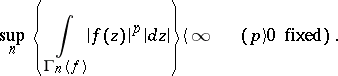This definition was proposed by M.V. Keldysh and M.A. Lavrent'ev , and is equivalent to V.I. Smirnov's definition  in which curves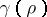are used instead of. These curves are the images of the circles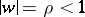under some univalent conformal mapping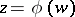from the disconto the domain, and the supremum is taken over all.

The classesare the best known and most thoroughly studied generalization of the Hardy classes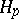, and are connected with them by the following relation:if and only if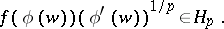The properties of the classesare closest to those ofin the case whenis a Smirnov domain. They have been generalized to domainswith boundaries of finite Hausdorff length. See also Boundary properties of analytic functions.

How to Cite This Entry:
Smirnov class. Encyclopedia of Mathematics. URL: http://encyclopediaofmath.org/index.php?title=Smirnov_class&oldid=14423
This article was adapted from an original article by E.P. Dolzhenko (originator), which appeared in Encyclopedia of Mathematics - ISBN 1402006098. See original article